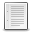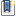File : Details

# Drawing Bezier Splines

drawing cubic bezier splines in the 2D-plane
Details
Version
1.0
Author
Andreas Geissler
Maintainer
Andreas Geissler
Categories
Supported Scilab Version
4.1
Creation Date
November 25, 2013Description
```            bezier - Cubic Bezier Spline (Interpolation)

Calling Sequence : zi = bezier(z,NPOI,modus)

Parameters

z : vector with complex values, which contains the so called weighing points.

NPOI : number of interpolating values. Default value is 40.

modus : a string, which specifies, if curves are closed ('c' or 'close') or
open       ('o' or 'open'). The default is 'close'.

zi : cubic bezier spline represented as a vector with complex elements

Description

If the weighing points are not given as complex vector, it is possible to
specify them by clicking with the mouse in a graphics window. If N weighing
points are given, the interpolation result has N*(NPOI-1) values when
modus='closed'. If modus='open', then the interpolation result has
(N-2)*(NPOI-1) values.

Example:

z=rand(1,10,'normal') + %i .*rand(1,10,'normal');
zi=bezier(z,10,'open');
plot2d(real(z),imag(z),style=-3);
plot2d(real(zi),imag(zi),style=2);
xclick();
xbasc();
zi=bezier();            ```Files (2)Screenshot
```Screenshot of the figure, which is generated by a call zout=bezier()
```Miscellaneous file
```Scilab function to define the cubic bezier spline
```News (0)Comments (0)Leave a comment
Login withEmail notifications